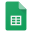# Calculus 1Thailand

## Lectures

Lecture 1 • Linear Equations in One Variable - PDF

Lecture 2 • Modeling with Linear Equations

Lecture 3 • Complex Numbers

Lecture 5 • Modeling with Quadratic Equations

Lecture 6 • Rational Equations

Lecture 7 • Linear Inequalities

Lecture 9 • Rational Inequalities

Lecture 10 • Absolute Value Inequalities

Lecture 11 • Systems of Linear Equations in Two Variables - PDF

Lecture 12 • Systems of Linear Equations in Three Variables

Lecture 13 • Modeling with Linear Systems

Lecture 14 • Matrix Solution, Partial Fractions, Linear Programming- PDF

## Homework

Homework 1 • 14 & 16, 18 & 20, 22 & 24, 26 & 28, 32 & 34 - Linear Equations

Homework 2 • 16, 22, 30, 36, 42 - Modeling with Linear Equations

Homework 3 • 32 & 34, 42 & 44, 58 & 60, 74, 90 & 98 - Complex Numbers

Homework 4 • 14 & 20, 28 & 32, 42 & 48, 60, 82 - Quadratic Equations

Homework 5 • 12 & 18, 20 & 22, 28, 42, 54 - Modeling with Quadratic Equations

Homework 6 • 20, 24, 32, 40, 42 - Rational Equations

Homework 7 • 18 & 20, 22 & 24, 26 & 28, 34, 38 - Linear Inequalities

Homework 8 • 44, 46, 48, 50, 52 - Quadratic Inequalities

Homework 9 • 62, 68, 72, 74, 78 - Rational Inequalities

Homework 10 • 28, 40, 66, 78, 80 - Absolute Value Inequalities

Homework 11 • 14, 16, 24, 26, 30 - Systems of Linear Equations

Homework 12 • 100, 102, 104, 106, 108 - Systems of Linear Equations

## ResultsResults / Calculus1 / Thailand

* The percentage of your homework is calculated as the maximum percentage times the ratio of your score to the maximum score

** In-class participation takes into account your class attendance and involvement in the process

## Literature

1. Precalculus, Margaret L. Lial, John Hornsby, David I. Schneider, Callie J. Daniels, Pearson 2016

2. Calculus I with Precalculus, Ron Larson, Robert P. Hostetler, Bruce H. Edwards, Cengage Learning 2011

3. Precalculus, Michael Sullivan, Pearson 2017

4. Introduction to Algebra, Richard Rusczyk, The Art of Problem Solving 2007